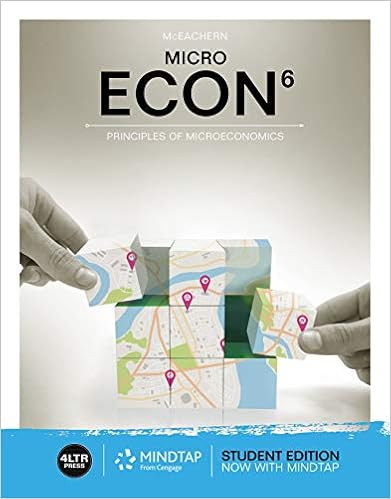# 86 assuming there is no profit markup on material

• 77
• 94% (52) 49 out of 52 people found this document helpful

This preview shows page 45 - 47 out of 77 pages.

##### We have textbook solutions for you!
The document you are viewing contains questions related to this textbook.The document you are viewing contains questions related to this textbook.
Chapter 9 / Exercise 8
ECON MICRO
McEachernExpert Verified
86. Assuming there is no profit markup on material cost, the amount to be added to each dollar of material cost to obtain the total material charge is: A. \$0.06. B. \$0.08. C. \$0.10. D. \$0.13. E. None of the answers is correct. Feedback A: This amount is incorrect. Feedback B: Correct! \$15,000 ÷ \$187,500 = \$0.08 Feedback C: This amount is incorrect. Feedback D: This amount is incorrect. Feedback E: This answer is wrong, because there is a correct answer choice listed. AACSB: Analytic AICPA BB: Critical Thinking AICPA FN: Measurement Blooms: Apply Difficulty: 3 Hard Learning Objective: 15-09
87. If a particular job takes 20 hours of labor and \$800 of materials, the price charged for the job is:
AACSB: Analytic AICPA BB: Critical Thinking AICPA FN: Measurement Blooms: Apply Difficulty: 3 Hard Learning Objective: 15-09 Feedback A: This amount is incorrect. Feedback B: This amount is incorrect. Feedback C: This amount is incorrect. Feedback D: Correct! (\$ 36.50 36 .75 x 20 hr.)+ \$800 + (800 x \$0.08) = \$1,59 4 9 Feedback E: This answer is wrong, because there is a correct answer choice listed. 15-45
##### We have textbook solutions for you!
The document you are viewing contains questions related to this textbook.The document you are viewing contains questions related to this textbook.
Chapter 9 / Exercise 8
ECON MICRO
McEachernExpert Verified
Chapter 15 - Target Costing and Cost Analysis for Pricing Decisions 88. Consider the following statements about competitive bidding: I. The higher the price that a company bids, the greater the profit if the firm gets the contract. II. Bidding a higher price increases the probability of obtaining a contract. III. A company that bids low to ensure acceptance of a contract may actually wind up bidding too low to make an acceptable profit. Which of the above statements is (are) true?
AACSB: Reflective Thinking AICPA BB: Marketing AICPA FN: Decision Making Blooms: Understand Difficulty: 2 Medium Learning Objective: 15-10 Feedback A: While this statement is true, there is a better answer choice. Feedback B: This statement is incorrect. Feedback C: While this statement is true, there is a better answer choice. Feedback D: Correct! Both statements I and III are true. Feedback E: One of these statements is incorrect. 89. If a company has excess capacity, which of the following is a sensible bidding strategy?
AACSB: Reflective Thinking AICPA BB: Marketing AICPA FN: Decision Making Blooms: Remember Difficulty: 1 Easy Learning Objective: 15-10 Feedback A: This bidding strategy is incorrect. Feedback B: Correct! This is the correct bidding strategy. Feedback C: This bidding strategy is incorrect. Feedback D: This bidding strategy is incorrect. Feedback E: This bidding strategy is incorrect.
•••